# Let's Get Started

Let's use what you know about y-intercepts to make predictions about how a change will affect the y-intercept of the function that describes that situation.

TEKS Standards and Student Expectations

A(3) Linear functions, equations, and inequalities. The student applies the mathematical process standards when using graphs of linear functions, key features, and related transformations to represent in multiple ways and solve, with and without technology, equations, inequalities, and systems of equations. The student is expected to:

A(3)(E) determine the effects on the graph of the parent function f(x) = x when f(x) is replaced by af(x), f(x) + d, f(x - c), and f(bx) for specific values of a, b, c, and d

Resource Objective(s)

Use graphs, tables, and equations to understand how changes in problem situations affect the y-intercept.

Essential Questions

How can you tell if a situation will increase or decrease the y-intercept?

How can you identify the y-intercept in a table if one of the ordered pairs is not (0, y)?

How can you determine the changes in the y-intercepts from an equation in slope-intercept form, y = mx + b?

Vocabulary

# Introduction

Linear functions can appear in a variety of representations.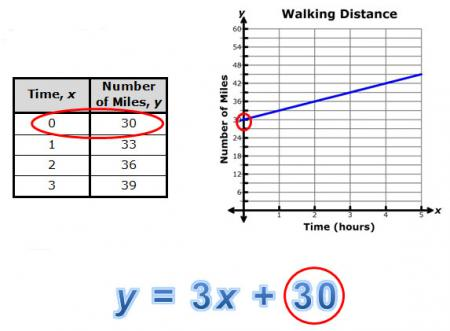Regardless of the representation, you can determine the coordinates of the y-intercept. If the table, graph, equation, or set of data describes a problem situation, you can interpret the y-intercept.

In the examples shown, the y-intercept, (0, 30) can be interpreted as the number of miles walked before the data was collected.In other words, the person had already walked a total of 30 miles.

We'll know to use what you know about y-intercepts to make predictions about how a change in the situation will affect the y-intercept of the function.

# Interpreting and Predicting Changes in y-Intercepts from a Graph

In previous resources, you learned how changes in m, the slope, affects, the steepness of the graph of y = mx +b  and changes in b, the y-intercept, affects where this function cross the y-axis."

Bethany received $30 from her favorite aunt for her birthday. She wants to save her money to purchase an e-reader. She decided to save the$30 and add \$5 from her own money each week. The amount of money she has saved as a function of time is shown in the graph below.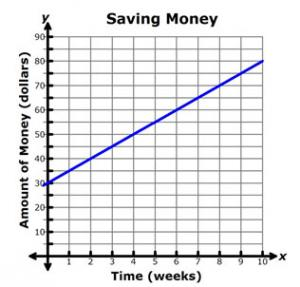Use the Saving Money graph to answer the next two questions.

Bethany wants to consider several different savings options. In the following interactive, match each graph with the situation that best describes what you notice about the y-intercept.

# Interpreting and Predicting Changes in y-Intercepts from a TableYou can also use a table of values to interpret and make predictions about the changes in the y-intercepts of linear functions.

For example, an auto mechanic charges a fixed service fee, an hourly rate for labor, and the cost of parts for a vehicle repair.

Yoko’s Auto and Body Shop uses a table like the one shown here to determine how much to charge for labor.

 Time               (hours, x) Labor Charge (dollars, y) 0.5 87.5 1 110 1.5 132.5 2.25 166.25 3 200

After reviewing the accounts, Yoko decides that he needs to increase the fixed service charge. Dwayne, his business partner, has prepared the tables below for Yoko to review.

# Interpreting and Predicting Changes in y-Intercepts from an Equation

Another representation commonly used for linear relationships is an equation or symbolic representation.Tami and her family went hiking in the Enchanted Rock State Natural Area, near Llano, Texas. The base of Enchanted Rock is 1,400 feet above sea level, and the top of Enchanted Rock is 425 feet above the ground. Enchanted Rock is one of the largest batholiths (underground rock formations) that has been uncovered by surface level erosion in the United States.

Tami used the following equation to describe her family’s elevation above sea level, y, as a function of time since the hike began, x.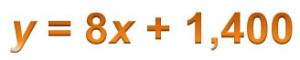# Summary

We've investigated how to interpret changes in y-intercepts for linear functions using graphs, tables, and equations.

Consider the graphs, equations, and tables shown below. Each pair of representations compares draining a pool with 12 feet of water at a rate of 1.5 feet per hour to draining a pool with the same shape and 9 feet of water at the same rate.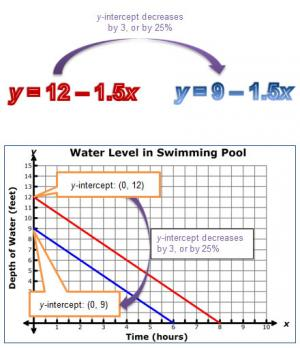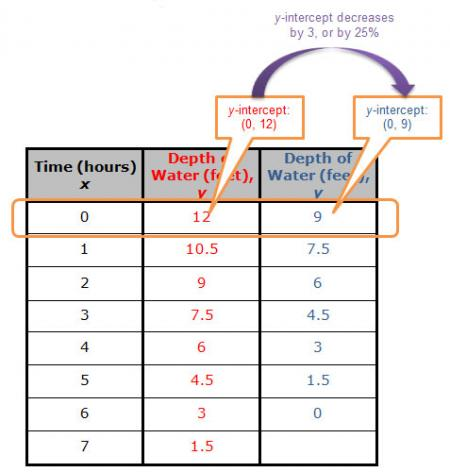In each representation, the y-intercept begins at (0, 12) and shows a decrease of 3 feet, or 25% from the original value of 12 feet, to a y-intercept of (0, 9).